TEL : +81 48 254 1744

"Easy" Gear Selection Screen

- Choose a gear by pitch, # of teeth, or bore size.
- 2D/3D Drawings Available
- PDF Catalog Available
- Strength Calculation Available

Go to Gear Selection Screen

CLOSE

# Gear Forces

Related links :
Free Gear Calculator

When the gear mesh transmits power, forces act on the gear teeth. As shown in Figure 12.1, if the Z-axis of the orthogonal 3-axes denotes the gear shaft, forces are defined as follows:
The force that acts in the X-axis direction is defined as the tangential force Ft (N)
The force that acts in the Y-axis direction is defined as the radial force. Fr (N)
The force that acts in the Z-axis direction is defined as the axial force Fx (N) or thrust .
Analyzing these forces is very important when designing gears. In designing a gear, it is important to analyze these forces acting upon the gear teeth, shafts, bearings, etc.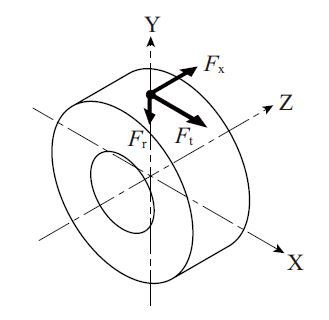Fig. 12.1 Direction of Forces Acting on a Gear

Table 12.1 presents the equations for forces acting on gears. The unit of torque T and T1 is N・m.

Table 12.1 Forces Acting upon a Gear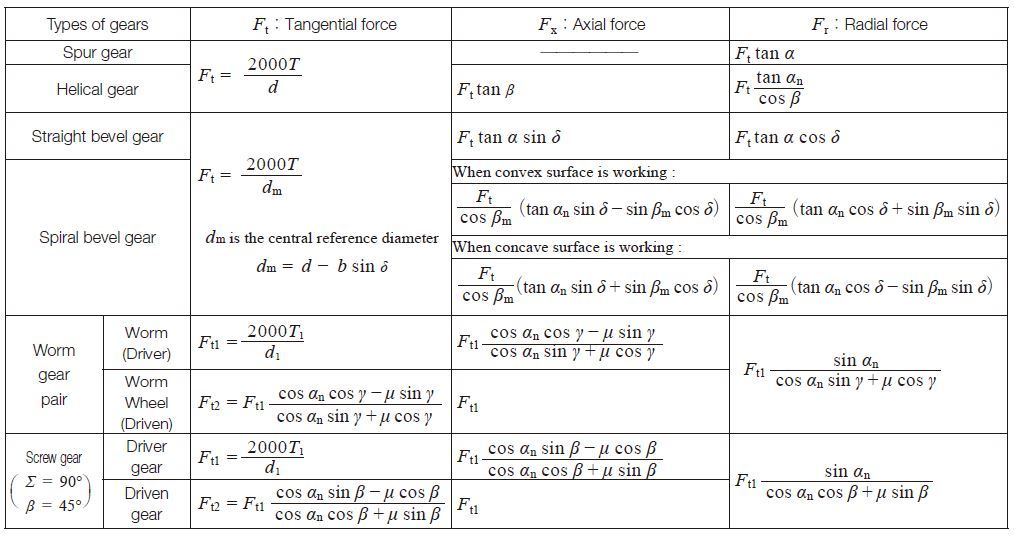## 12.1 Forces in a Parallel Axis Gear Mesh

Figure 12.2 shows forces that act on the teeth of a helical gear . Larger helix angle of the teeth, has larger thrust (axial force). In case of spur gears , no axial force acts on teeth.

Fig.12.2 Direction of Forces acting on a Helical Gear Mesh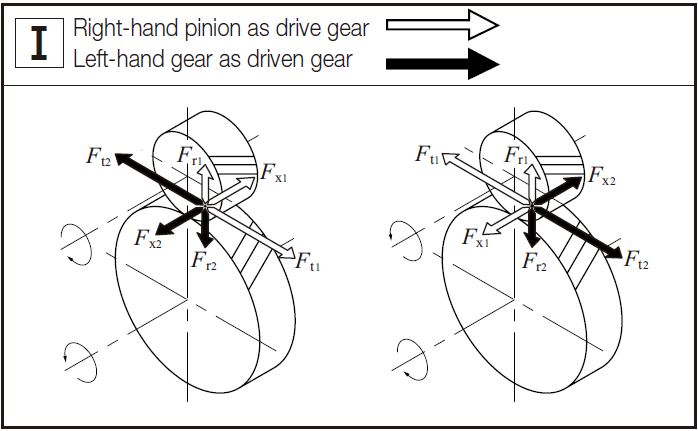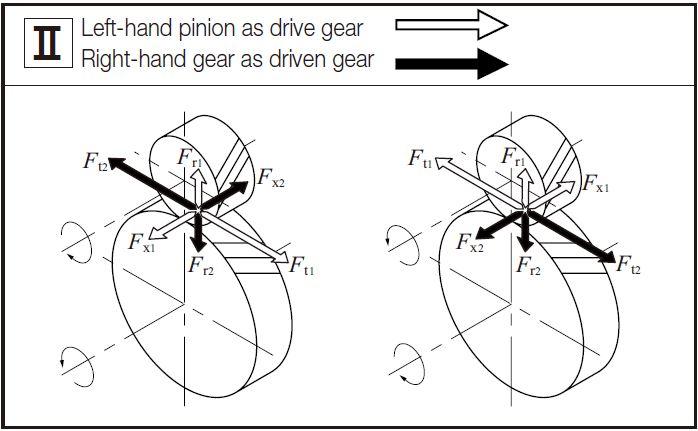Table 12.2 Calculation Examples (Spur Gear)

Number Specification Symbol Unit Formula Spur Gear
Pinion Gear
1 Module m mm Set Value 2
2 Normal Pressure Angle αn mm Set Value 2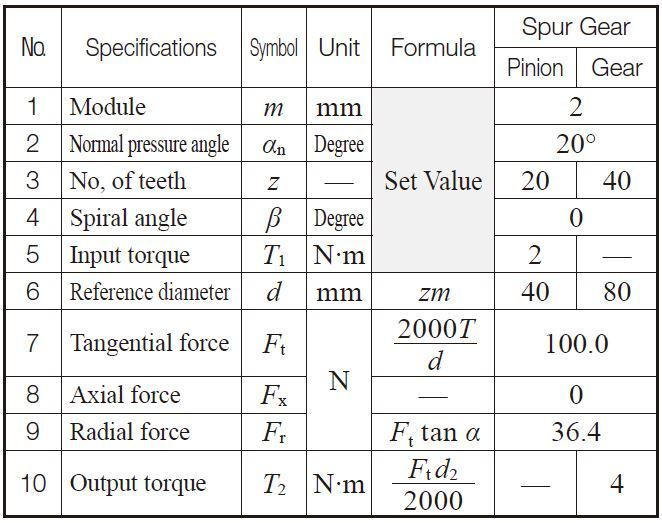## 12.2 Forces in an Intersecting Axis Gear Mesh

In the meshing of a pair of bevel gears with shaft angle Σ= 90 degree, the axial force acting on drive gear equals the radial force acting on driven gear. Similarly, the radial force acting on drive gear equals the axial force acting on driven gear.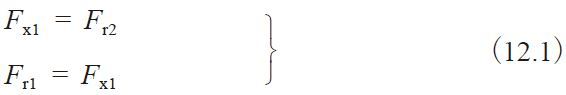(1) Forces in a Straight Bevel Gear Mesh
Figure 12.3 shows how forces act on a straight bevel gear mesh.

Fig 12.3 Directions of Forces acting on a Straight Bevel Gear Mesh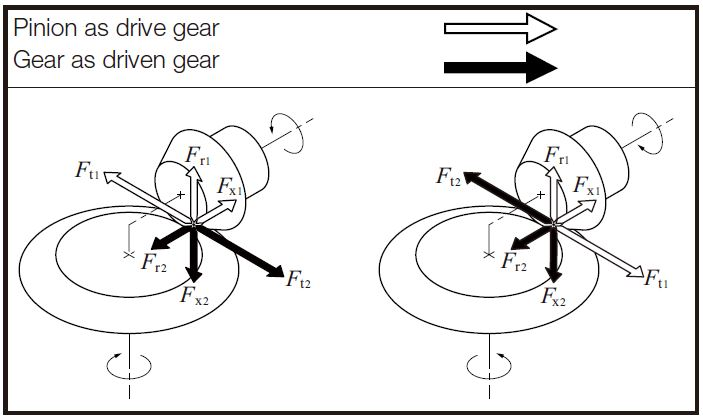(2) Forces in a Spiral Bevel Gear Mesh
Spiral bevel gear teeth have convex and concave sides. Depending on which surface the force is acting on, the direction and magnitude changes. They differ depending upon which is driver and which is the driven. Table 12.5 shows the meshing tooth face.
Figure 12.5 shows how the forces act on the teeth of a spiral bevel gear mesh. Negative axial force is the thrust, pushing the two gears together. The bearing must be designed carefully so that it can receive this negative thrust. If there is any axial play in the bearing, it may lead to the undesirable condition of the mesh having no backlash.

Table 12.3 Calculation Examples (Spiral Gear)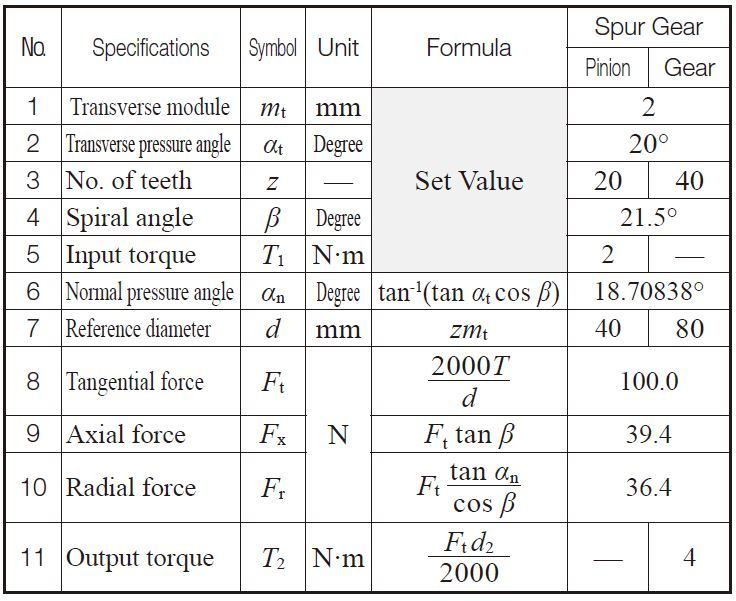Table 12.4 Calculation Examples (Straight Bevel Gear)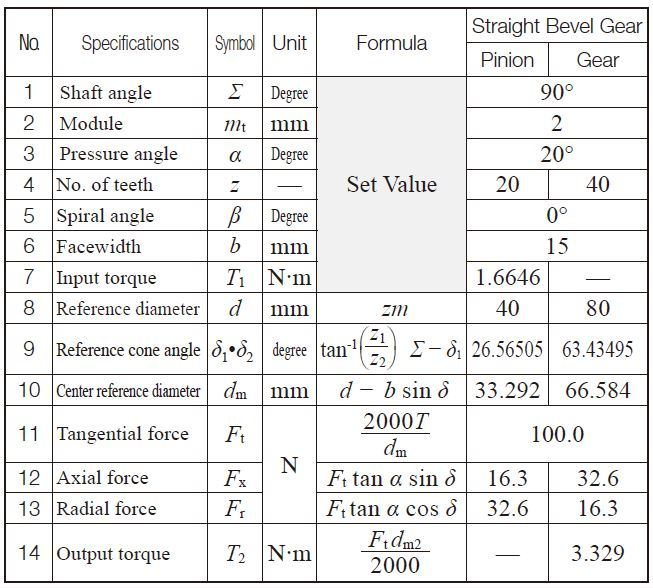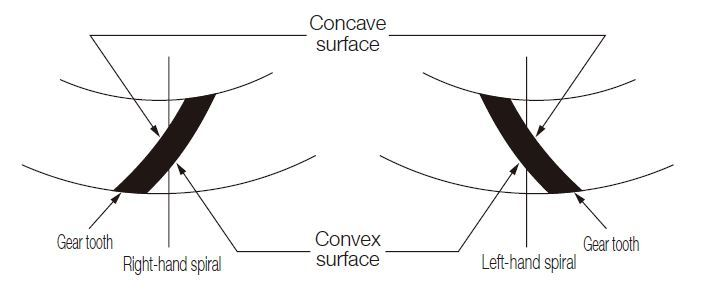Fig.12.4 Convex surface and concave surface of a spiral bevel gear

Table 12.5 Meshing Tooth Face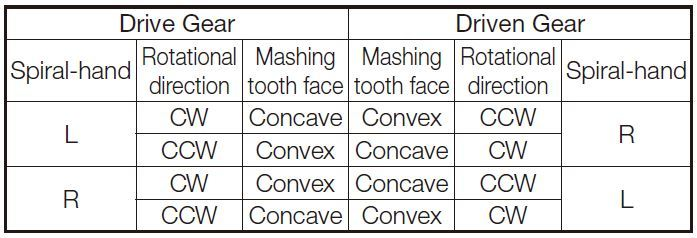Fig.12.5 The Directions of Forces Carried by Spiral Bevel Gears
Σ = 90°, αn = 20°, βm = 35°, z2 / z1 < 1.57357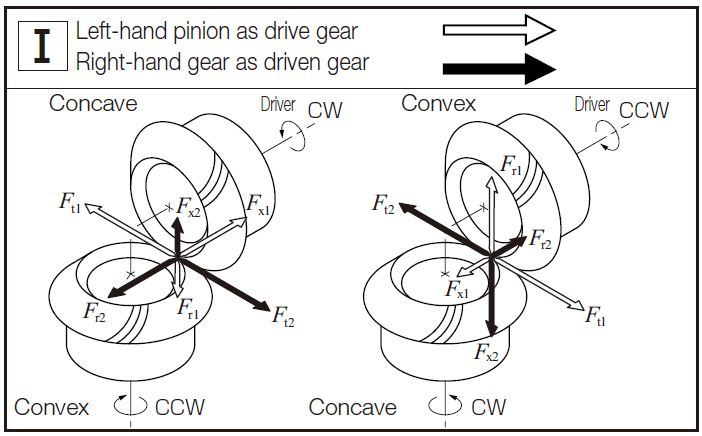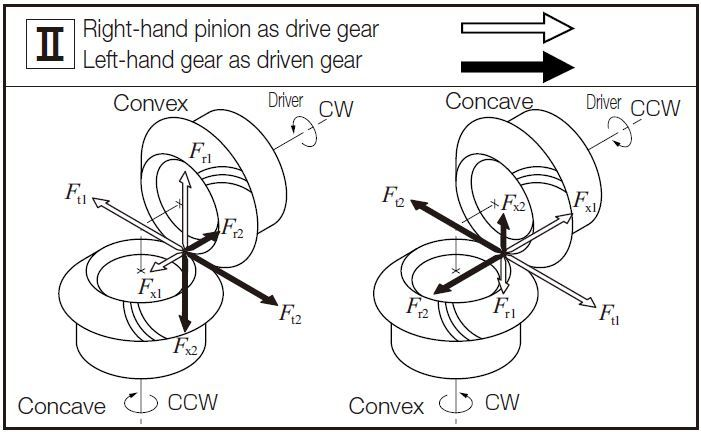Σ = 90°, αn = 20°, βm = 35°, z2 / z1 ≧ 1.57357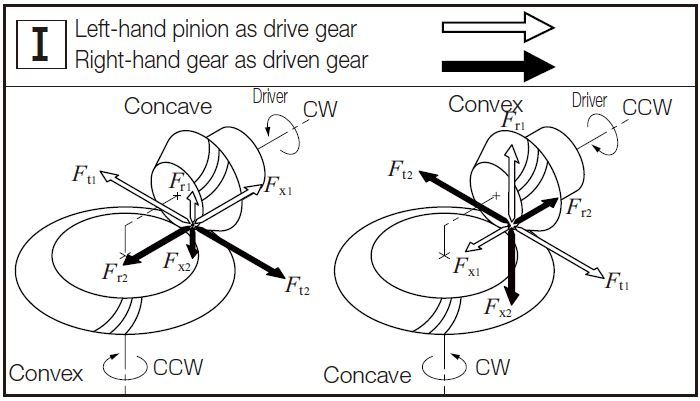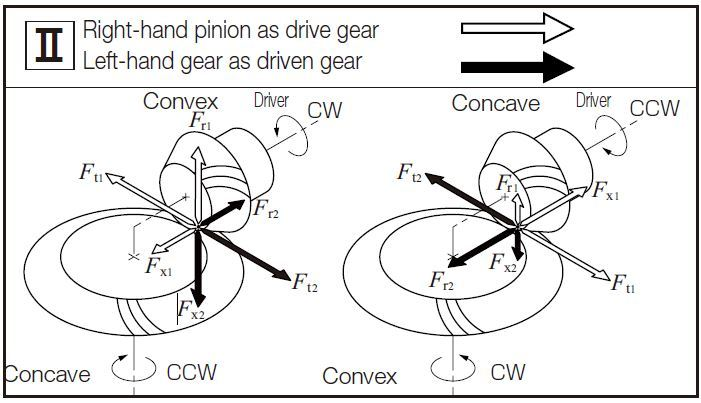Table 12.6 Calculation Examples (Spiral Bevel Gears)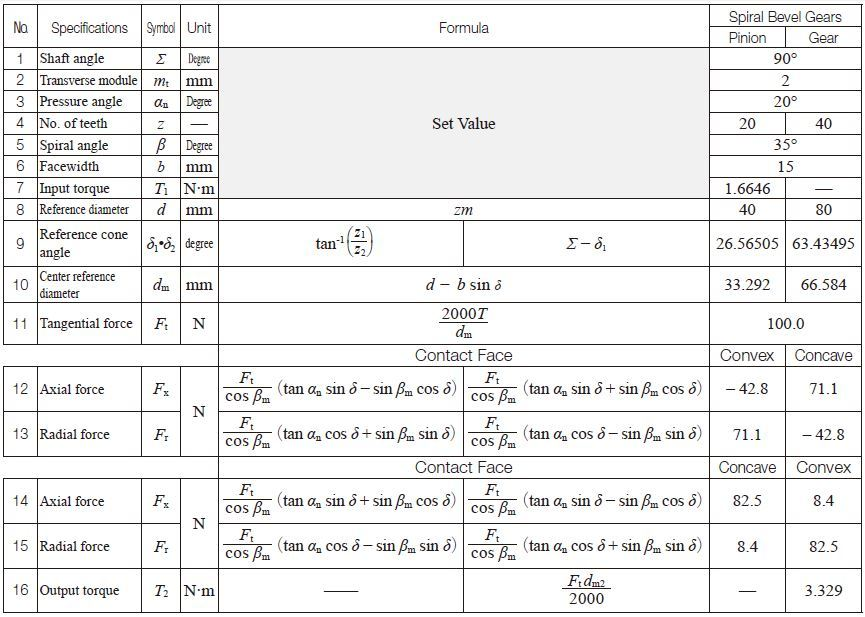## 12.3 Forces in a Nonparallel and Nonintersecting Axes Gear Mesh

(1) Forces in a Worm Gear Pair Mesh
Figure 12.6 shows how the forces act on the teeth of a worm gear pair mesh with a shaft angle Σ = 90 degree. Since the power transmission of the worm gear pair mesh has a sliding contact nature, the coefficient of friction on the tooth surface has a great effect on the transmission efficiency ηR and the force acting on the gear mesh.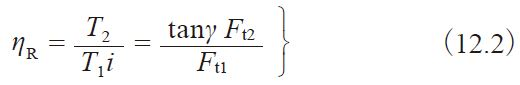Fig. 12.6 Direction of Forces in a Worm Gear Pair Mesh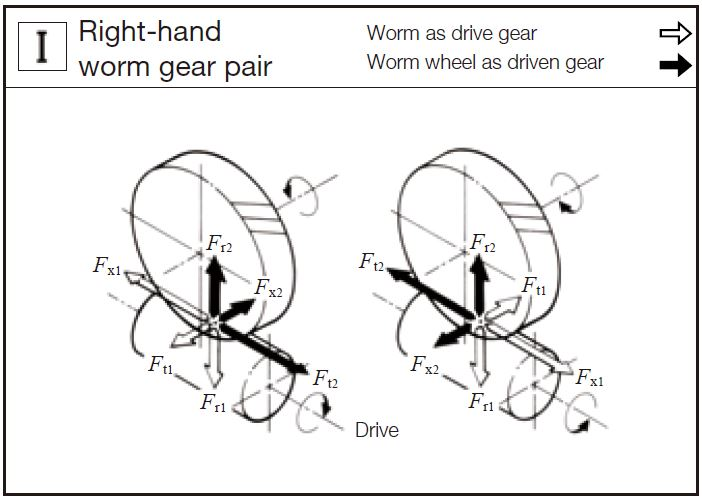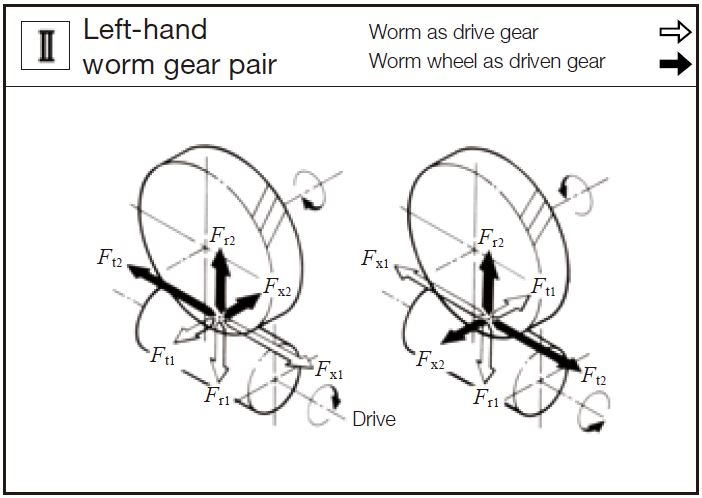Table 12.7 Calculation Examples (Worm Gear Pair)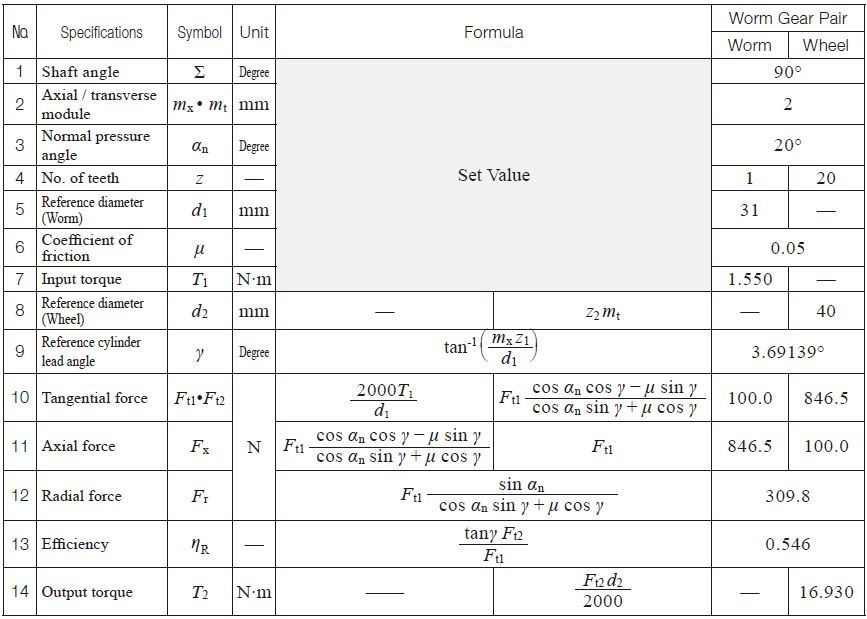(2) Forces in a Screw Gear Mesh
The forces in a screw gear mesh are similar to those in a worm gear pair mesh.
Figure 12.7 shows the force acts on the teeth of a screw gear mesh with a shaft angle Σ = 90 degree, a helix angle = 45 degree.

Fig 12.7 Direction of Forces in a Screw Gear Mesh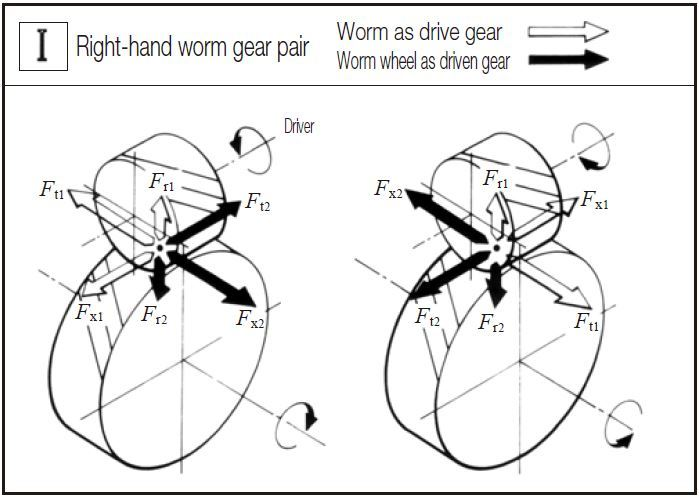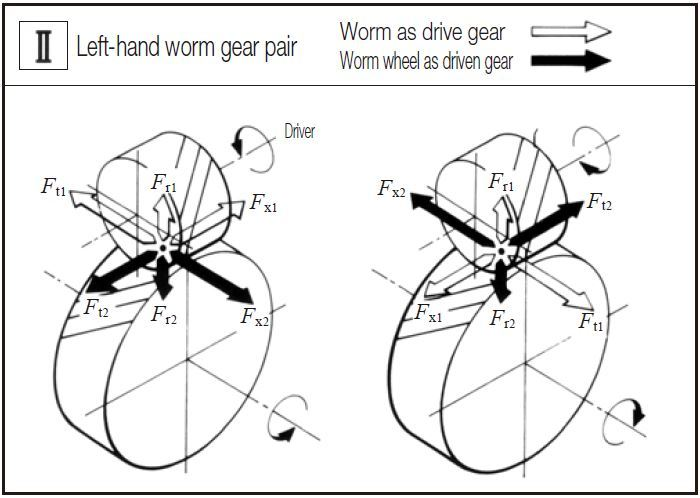Table 12.8 Calculation Examples (Screw Gear)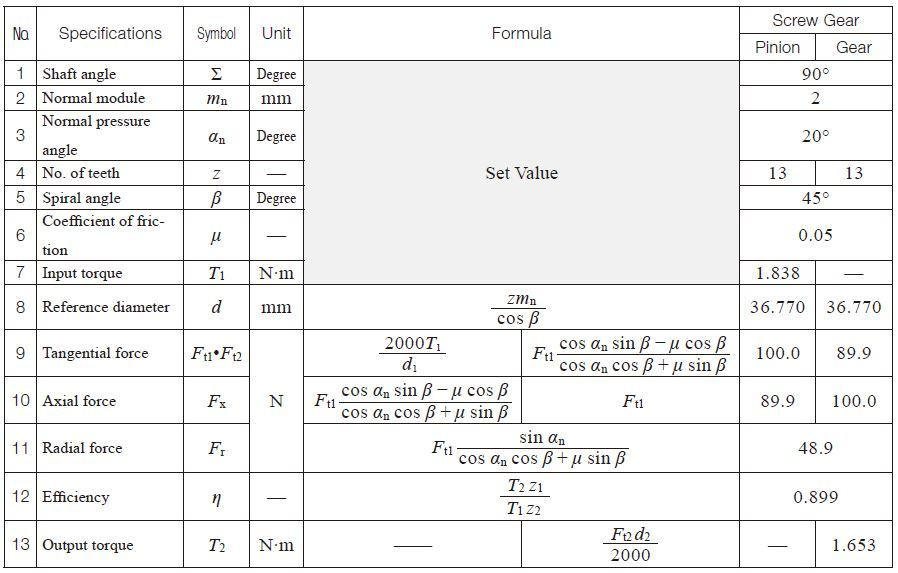Related links :
Know about gear transmission torque
Gear Rack and Pinion

Go to Top